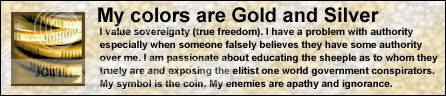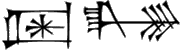## Recommended Posts

I have been making a Scorched Earth clone in auto it for the last few days. I've got 500 lines of code just for the GUI and to load the INI files.

Anyway things were going great until i noticed that it treats my \$Current_Player variable as a Local Variable instead of a Global Variable.

I know that most of my code has got to be confusing but whatever.

The problem occurs in this loop within the Main() Function. Notice that when you are in the function "Buy," \$Current_Player = 0. The title of the Buy window should include the proper player name. Also each player should start with \$200, which is enough to buy a "fourth of july" weapon.

for \$Current_Player = 0 to \$Active_Players-1

\$Inventory[\$Current_Player] = 666

\$Player_Stats[\$Current_Player] = InputBox("Playa " & \$Current_Player+1 & " Name","Whas 'dis playa's name dawg!","bitch " & \$Current_Player+1,"",100,130)

\$Player_Stats[\$Current_Player] = 0

\$Player_Stats[\$Current_Player] = 0

\$Player_Stats[\$Current_Player] = 100

\$Player_Stats[\$Current_Player] = 100

\$Player_Stats[\$Current_Player] = 1

\$Player_Stats[\$Current_Player] = \$pi/2

\$Player_Stats[\$Current_Player] = 100

\$Player_Stats[\$Current_Player] = 0

\$Player_Stats[\$Current_Player] = 1

\$Player_Stats[\$Current_Player] = 0

\$Player_Stats[\$Current_Player] = 200

\$Player_Stats[\$Current_Player] = 0

Next

If anybody can figure this out i will greatly appreciate it.

This is the INI file of constants and weapons. Yea, i know there are a lot of "Fourth of July" weapons... Save as "Data.ini" in the script directory

CODE
[Constants]

Types_of_Guns=18

Types_of_Terrain=2

[Terrain_0]

Name=Mountain

BK_Color=0x000000

Gravity=1

Ground_Color=0x00ff00

Wind_Init_Rand_Max=10

Wind_Init_Rand_Min=0

Wind_Rand_Max=.5

Wind_Rand_Min=0

Init_Height = 50

Sin1_Outer_Coeff=10

Sin2_Outer_Coeff=6

Sin3_Outer_Coeff=14

Sin4_Outer_Coeff=10

Sin1_Inner_Coeff=.2

Sin2_Inner_Coeff=.5

Sin3_Inner_Coeff=.1

Sin4_Inner_Coeff=.05

World_Width=200

[Terrain_1]

Name=Question

BK_Color=0x110000

Gravity=1

Ground_Color=0xff0000

Wind_Init_Rand_Max=10

Wind_Init_Rand_Min=0

Wind_Rand_Max=.5

Wind_Rand_Min=0

Init_Height = 50

Sin1_Outer_Coeff=2

Sin2_Outer_Coeff=6

Sin3_Outer_Coeff=7

Sin4_Outer_Coeff=8

Sin1_Inner_Coeff=.2

Sin2_Inner_Coeff=.5

Sin3_Inner_Coeff=.1

Sin4_Inner_Coeff=.05

World_Width=100

[Weapon_index#]

name=

cost=

accuracy=Radians, used in split as well as firing

cooldown=affected on tank

damage=damage at center

explosion_type=explosion type 0= linear 1= exponental 2= flat

split_time=after how many time does it split

split_number=splits into how many when

split_to_index#=refs annother bullet stat #

split_type=refs type of split true = spread, false = linear

[Weapon_0]

name=Bomb Jr

cost=0

accuracy=.2

cooldown=20

damage=20

explosion_type=0

split_time=-1

split_number=0

split_to_index#=0

split_type=false

[Weapon_1]

name=Fourth of July

cost=200

accuracy=.25

cooldown=25

damage=100

explosion_type=0

split_time=10

split_number=10

split_to_index#=0

split_type=true

[Weapon_2]

name=Fourth of July

cost=200

accuracy=.25

cooldown=25

damage=100

explosion_type=0

split_time=10

split_number=10

split_to_index#=0

split_type=true

[Weapon_3]

name=Fourth of July

cost=200

accuracy=.25

cooldown=25

damage=100

explosion_type=0

split_time=10

split_number=10

split_to_index#=0

split_type=true

[Weapon_4]

name=Fourth of July

cost=200

accuracy=.25

cooldown=25

damage=100

explosion_type=0

split_time=10

split_number=10

split_to_index#=0

split_type=true

[Weapon_5]

name=Fourth of July

cost=200

accuracy=.25

cooldown=25

damage=100

explosion_type=0

split_time=10

split_number=10

split_to_index#=0

split_type=true

[Weapon_6]

name=Fourth of July

cost=200

accuracy=.25

cooldown=25

damage=100

explosion_type=0

split_time=10

split_number=10

split_to_index#=0

split_type=true

[Weapon_7]

name=Fourth of July

cost=200

accuracy=.25

cooldown=25

damage=100

explosion_type=0

split_time=10

split_number=10

split_to_index#=0

split_type=true

[Weapon_8]

name=Fourth of July

cost=200

accuracy=.25

cooldown=25

damage=100

explosion_type=0

split_time=10

split_number=10

split_to_index#=0

split_type=true

[Weapon_9]

name=Fourth of July

cost=200

accuracy=.25

cooldown=25

damage=100

explosion_type=0

split_time=10

split_number=10

split_to_index#=0

split_type=true

[Weapon_10]

name=Fourth of July

cost=200

accuracy=.25

cooldown=25

damage=100

explosion_type=0

split_time=10

split_number=10

split_to_index#=0

split_type=true

[Weapon_11]

name=Fourth of July

cost=200

accuracy=.25

cooldown=25

damage=100

explosion_type=0

split_time=10

split_number=10

split_to_index#=0

split_type=true

[Weapon_12]

name=Fourth of July

cost=200

accuracy=.25

cooldown=25

damage=100

explosion_type=0

split_time=10

split_number=10

split_to_index#=0

split_type=true

[Weapon_13]

name=Fourth of July

cost=200

accuracy=.25

cooldown=25

damage=100

explosion_type=0

split_time=10

split_number=10

split_to_index#=0

split_type=true

[Weapon_14]

name=Fourth of July

cost=200

accuracy=.25

cooldown=25

damage=100

explosion_type=0

split_time=10

split_number=10

split_to_index#=0

split_type=true

[Weapon_15]

name=Fourth of July

cost=200

accuracy=.25

cooldown=25

damage=100

explosion_type=0

split_time=10

split_number=10

split_to_index#=0

split_type=true

[Weapon_16]

name=Fourth of July

cost=200

accuracy=.25

cooldown=25

damage=100

explosion_type=0

split_time=10

split_number=10

split_to_index#=0

split_type=true

[Weapon_17]

name=Fourth of July

cost=200

accuracy=.25

cooldown=25

damage=100

explosion_type=0

split_time=10

split_number=10

split_to_index#=0

split_type=true

[Weapon_18]

name=Fourth of July

cost=200

accuracy=.25

cooldown=25

damage=100

explosion_type=0

split_time=10

split_number=10

split_to_index#=0

split_type=true

[Weapon_19]

name=Fourth of July

cost=200

accuracy=.25

cooldown=25

damage=100

explosion_type=0

split_time=10

split_number=10

split_to_index#=0

split_type=true

[Weapon_20]

name=Fourth of July

cost=200

accuracy=.25

cooldown=25

damage=100

explosion_type=0

split_time=10

split_number=10

split_to_index#=0

split_type=true

[Weapon_21]

name=Fourth of July

cost=200

accuracy=.25

cooldown=25

damage=100

explosion_type=0

split_time=10

split_number=10

split_to_index#=0

split_type=true

[Weapon_22]

name=Fourth of July

cost=200

accuracy=.25

cooldown=25

damage=100

explosion_type=0

split_time=10

split_number=10

split_to_index#=0

split_type=true

[Weapon_23]

name=Fourth of July

cost=200

accuracy=.25

cooldown=25

damage=100

explosion_type=0

split_time=10

split_number=10

split_to_index#=0

split_type=true

[Weapon_24]

name=Fourth of July

cost=200

accuracy=.25

cooldown=25

damage=100

explosion_type=0

split_time=10

split_number=10

split_to_index#=0

split_type=true

[Weapon_25]

name=Fourth of July

cost=200

accuracy=.25

cooldown=25

damage=100

explosion_type=0

split_time=10

split_number=10

split_to_index#=0

split_type=true

[Weapon_26]

name=Fourth of July

cost=200

accuracy=.25

cooldown=25

damage=100

explosion_type=0

split_time=10

split_number=10

split_to_index#=0

split_type=true

[Weapon_27]

name=Fourth of July

cost=200

accuracy=.25

cooldown=25

damage=100

explosion_type=0

split_time=10

split_number=10

split_to_index#=0

split_type=true

[Weapon_28]

name=Fourth of July

cost=200

accuracy=.25

cooldown=25

damage=100

explosion_type=0

split_time=10

split_number=10

split_to_index#=0

split_type=true

##### Share on other sites

Remember, programs only do what the programmer tells it to do, so if AutoIt is acting retarded... well you do the math.

Agreement is not necessary - thinking for one's self is!##### Share on other sites

Never try to define the variable used for counting in a for...next loop outside of said loop. This counting variable is only meant to be temporary and you shouldn't ever rely on its existence after the loop exits, this is improper coding in any language.

```Global \$X

DoStuff()
MsgBox(0,"",\$X) ;Shows empty

Func DoStuff()
For \$X = 0 to 3
Next
EndFunc```
Edited by weaponx

##### Share on other sites

Remember, programs only do what the programmer tells it to do, so if AutoIt is acting retarded... well you do the math.

i know, look if you can find where i declare \$Current_Player as a local variable, then you can call me retarded all you want...

##### Share on other sites

Never try to define the variable used for counting in a for...next loop outside of said loop. This counting variable is only meant to be temporary and you shouldn't ever rely on its existence after the loop exits, this is improper coding in any language.

```Global \$X

DoStuff()
MsgBox(0,"",\$X) ;Shows empty

Func DoStuff()
For \$X = 0 to 3
Next
EndFunc```
why does it disappear though if it is a global variable?

damn my lazyness, i knew i was doing it wrong but i didn't think it would hurt anything in the end...

Edited by ToxicWaste55

##### Share on other sites

Thanks WeaponX, i never thought that could happen.

i have completely taught myself programming so nobody ever told me that before, and it is a bit illogical for the value not to carry over.

and FossilRock, i guess i was the retarded one, but since you didn't try to help and just attacked me instead, you're not really helping the autoit community.

##### Share on other sites

Thanks WeaponX, i never thought that could happen.

i have completely taught myself programming so nobody ever told me that before, and it is a bit illogical for the value not to carry over.

and FossilRock, i guess i was the retarded one, but since you didn't try to help and just attacked me instead, you're not really helping the autoit community.

He never said you are retarded. He said "so if AutoIt is acting retarded".

If the Terminator kills John Connor in the past, is it the Terminators fault, or is it future John Connor's fault for reprogramming the Model 101 using a For / Next loop and expecting the counting variable to remain viable globally?

##### Share on other sites

He never said you are retarded. He said "so if AutoIt is acting retarded".

If the Terminator kills John Connor in the past, is it the Terminators fault, or is it future John Connor's fault for reprogramming the Model 101 using a For / Next loop and expecting the counting variable to remain viable globally?

aww, whatever, i give up.

i was just ignorant.

i'll post the completed version of this, assuming i finish it, at some point

## Create an account

Register a new account

• ### Recently Browsing   0 members

×

• Wiki

• Back

• #### Beta

• Git
• FAQ
• Our Picks
×
• Create New...